# 算法图解 第7章 狄克斯特拉算法

• 继续图的讨论，介绍加权图------提高或降低某些边的权重。
• 介绍狄克斯特拉算法，让你能够找出加权图中前往X的最短路径。
• 介绍图中的环，它导致狄克斯特拉算法不管用。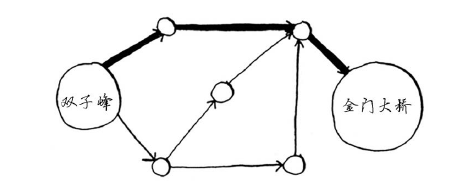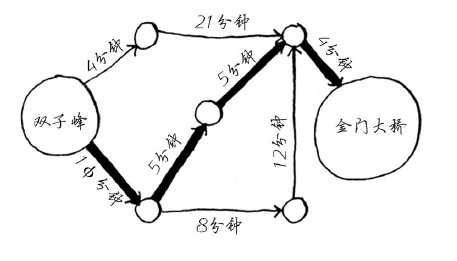## 7.1 使用狄克斯特拉算法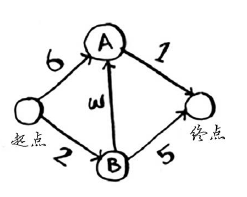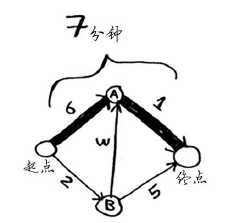（1）找出“最便宜”的节点，即可在最短时间内到达的节点。

（2）更新该节点的邻居的开销，其含义稍后介绍。

（3）重复这个过程，直到对图中的每个节点都这样做了。

（4）计算最终路径。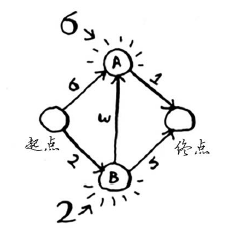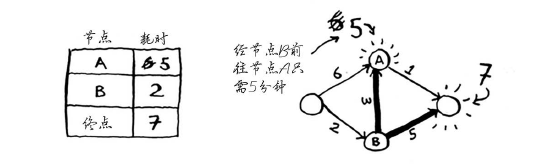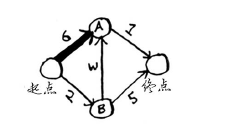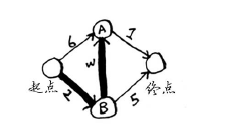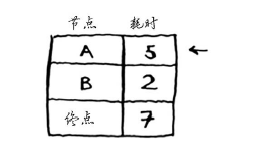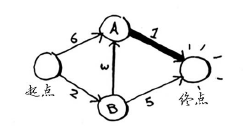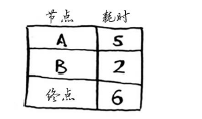## 7.2 术语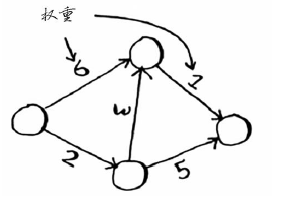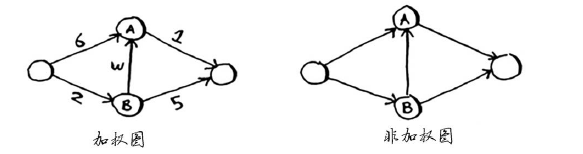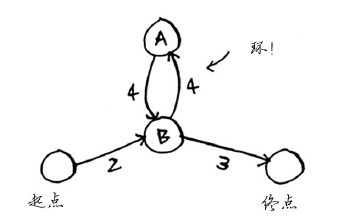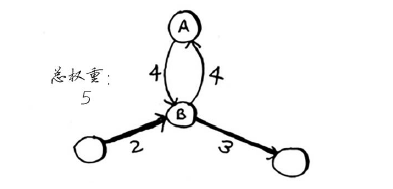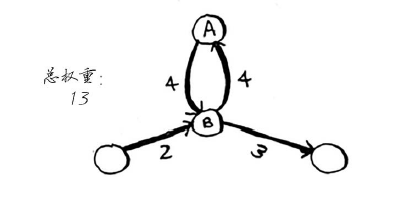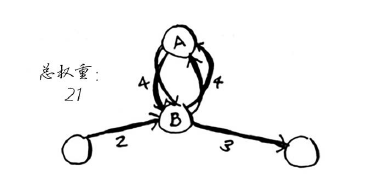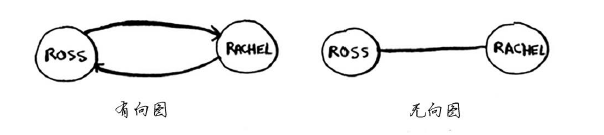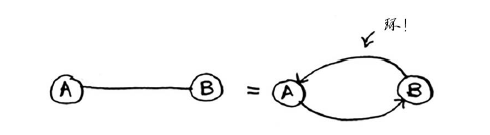## 7.3 换钢琴

Alex说：“这是我最喜欢的乐队Destroyer的海报，我愿意拿它换你的乐谱。如果你再加5美元，还可拿乐谱换我这张稀有的Rick Astley 黑胶唱片。"  Amy说:"哇，我听说这张黑胶唱片里有首非常好听的歌曲，我愿意拿我的吉他或架子鼓换这张海报或黑胶唱片。"

Beethoven惊呼：”我一直想要吉他，我愿意拿我的钢琴换Amy的吉他或架子鼓。“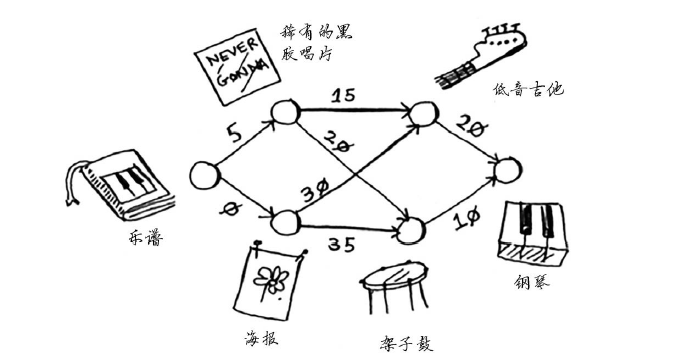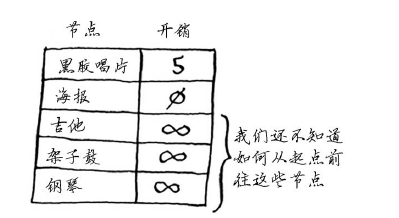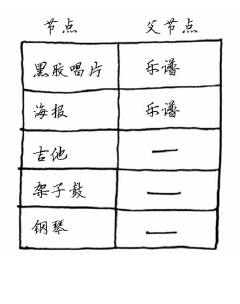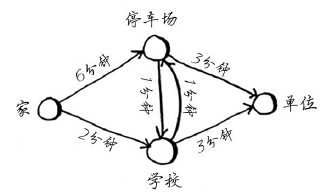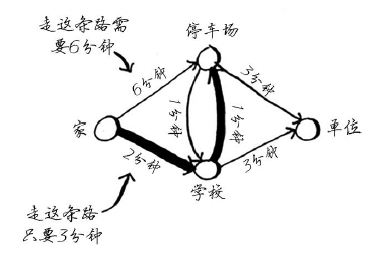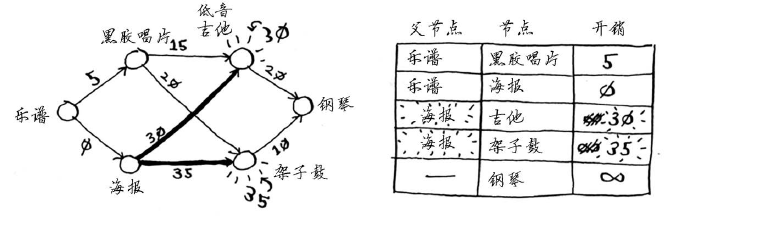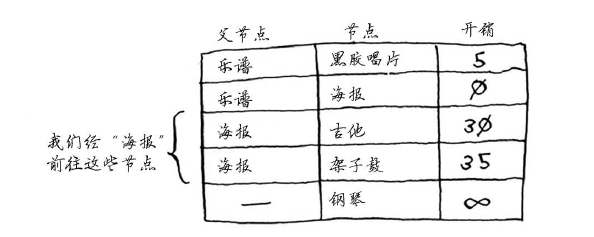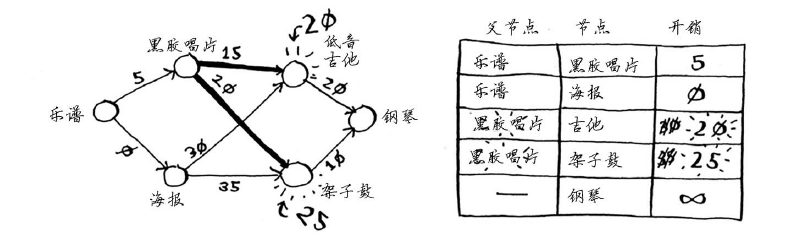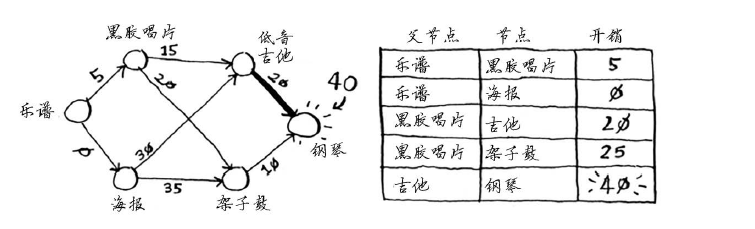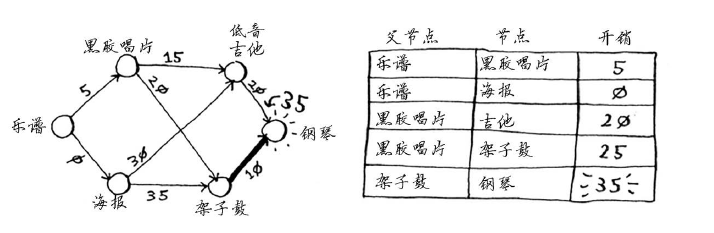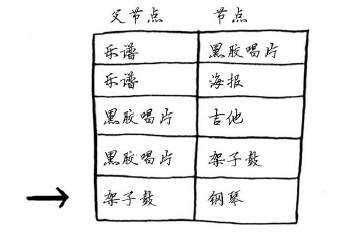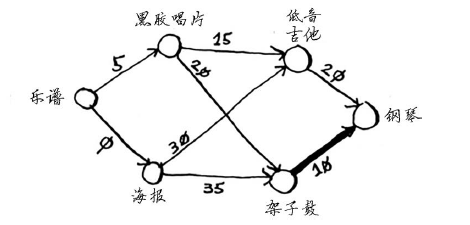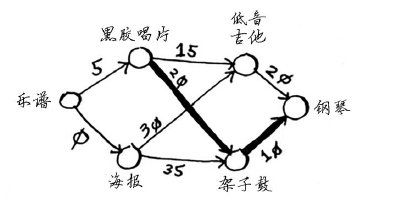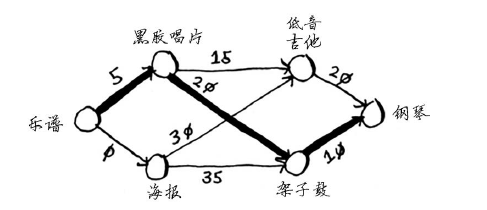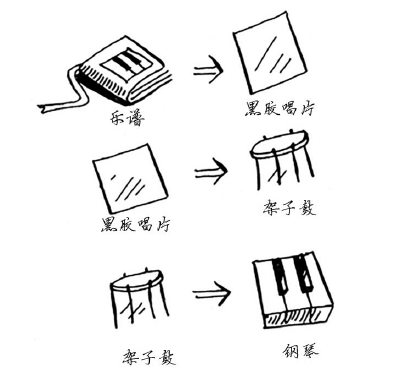## 7.4 负权边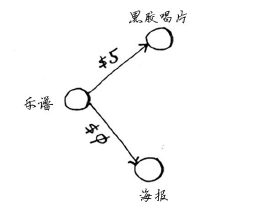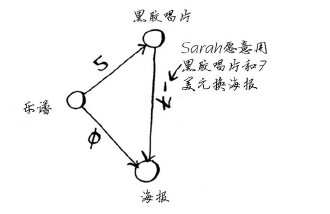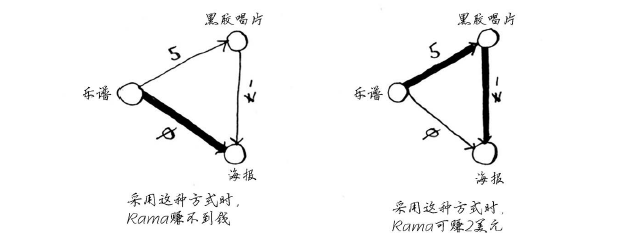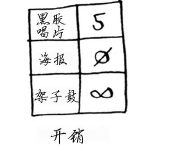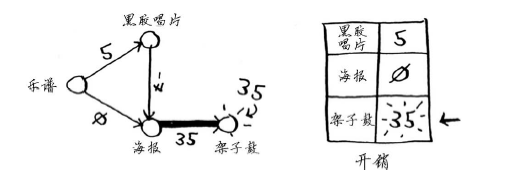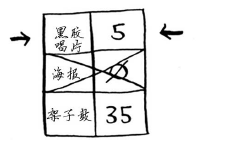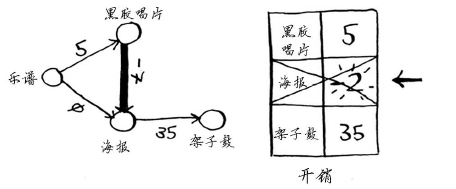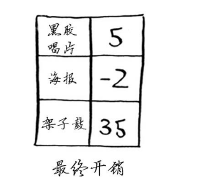7.5 实现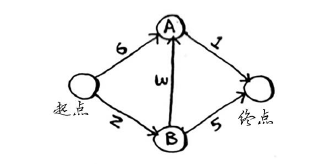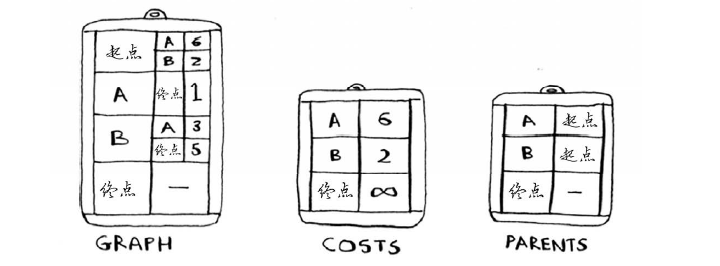graph = {}

graph["ypu"] = ["alice","bob","claire"]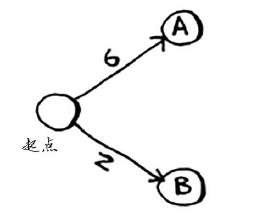graph["start"] = {}

graph["start"]["a"] = 6

graph["start"]["b"] = 2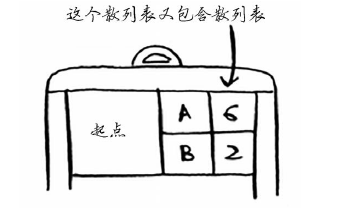>>> print graph["start"].keys()

["a","b"]

>>> print graph["start"]["a"]

2

>>> print graph["start"]["b"]

6

graph['a'] = {}

grapn["a"]["fin"] = 1

graph["b"] ={}

graph["b"]["a"] = 3

graphn["b"]["fin"] = 5

graph["fin"] = {}  <—————终点没有任何邻居。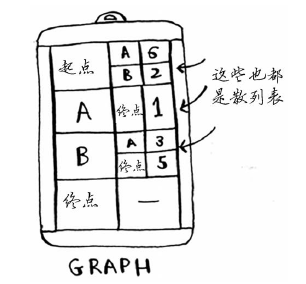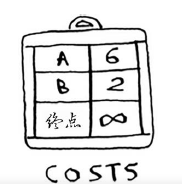infinity = float("inf")

infinity = float("inf")

consts = {}

const["a"] = 6

const["b"] = 2

consts["fin"] = infinity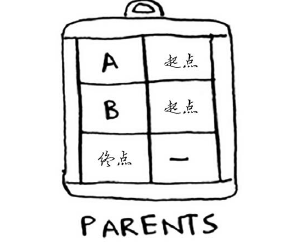parents = {}

parents["a"] = "start"

parents["b"] = "start"

parents["fin"] = None

processed = []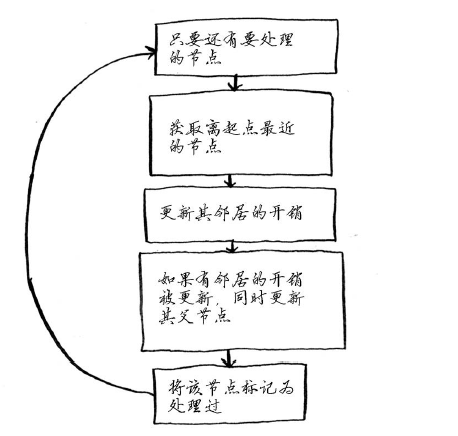#在未处理的节点中找出开销最小的节点
node = find_lowest_cost_node(consts)
while node is not None: #这个while循环在所有节点都被处理过后结束
const = consts[node]
neighbors = graph[node]
for n in neighbors.keys(): #遍历当前节点所有邻居
new_cost = cost + neighbors[n]
if const[n] > new_cost: #如果经当前节点前往邻居更近，
const[n] = new_cost #就更新该邻居的开销
parents[n] = node   #同时将该邻居的父节点设置为当前节点
processed.append(node)      #即当前节点标记为处理过
node = find_lowest_const_node(consts) #找出接下来要处理的节点，并循环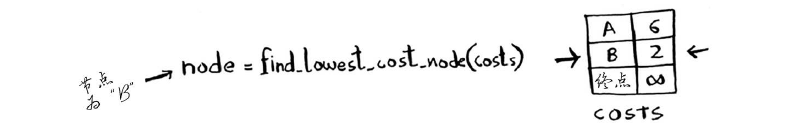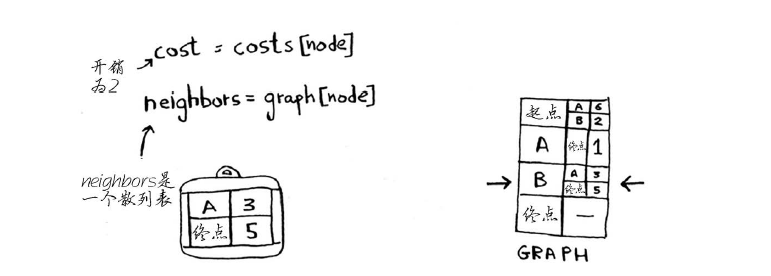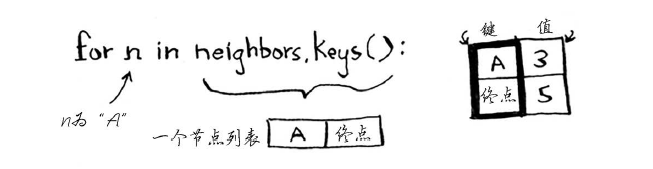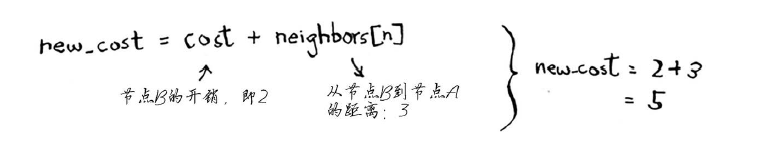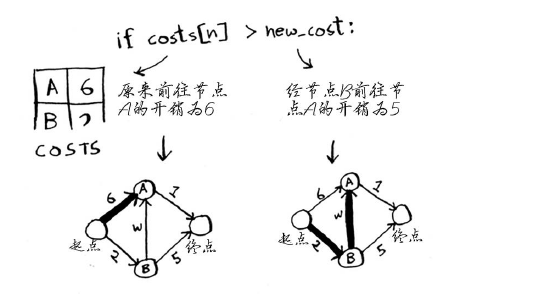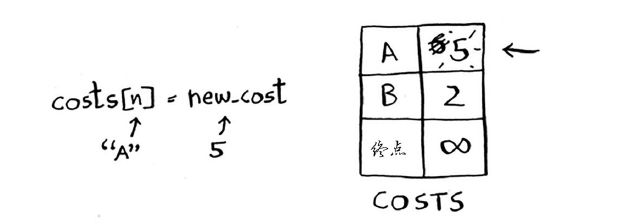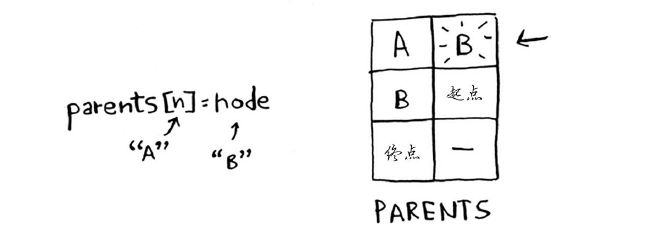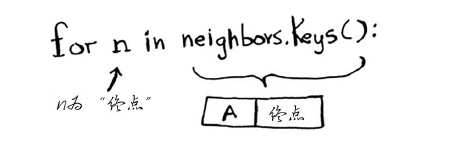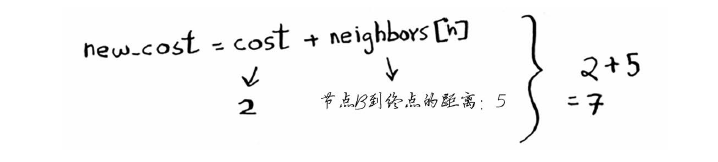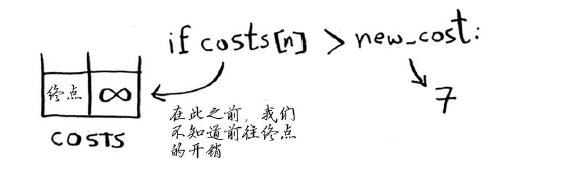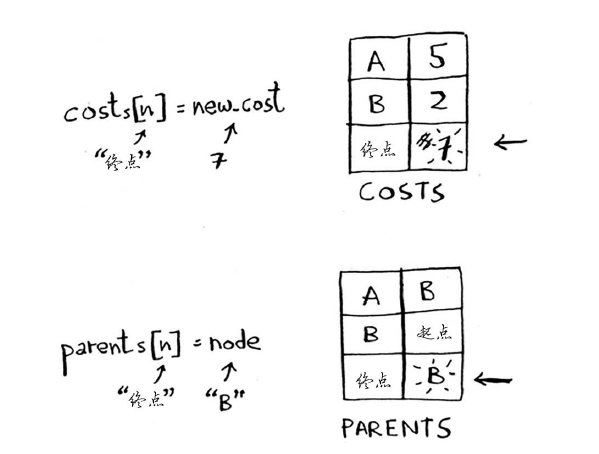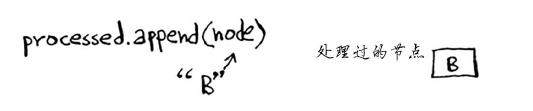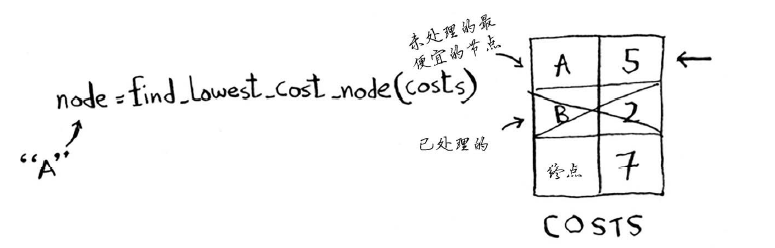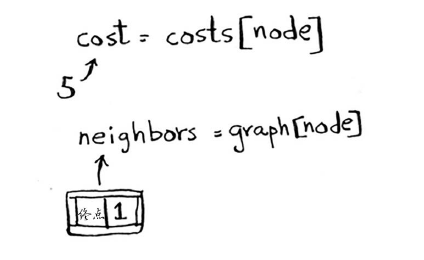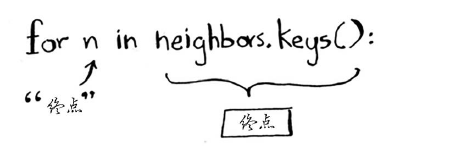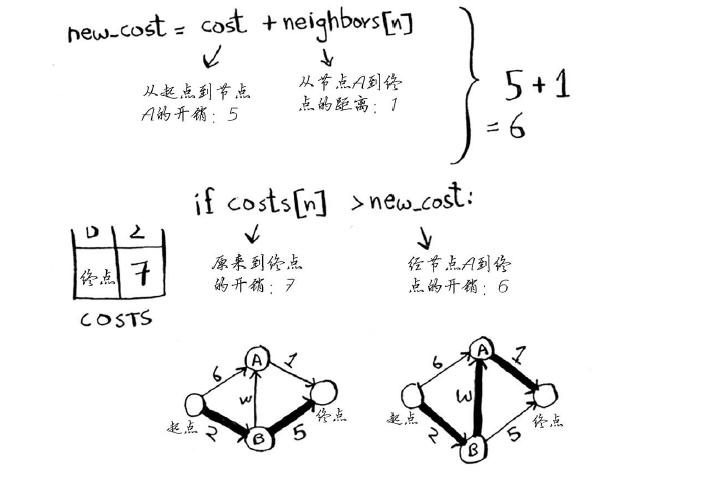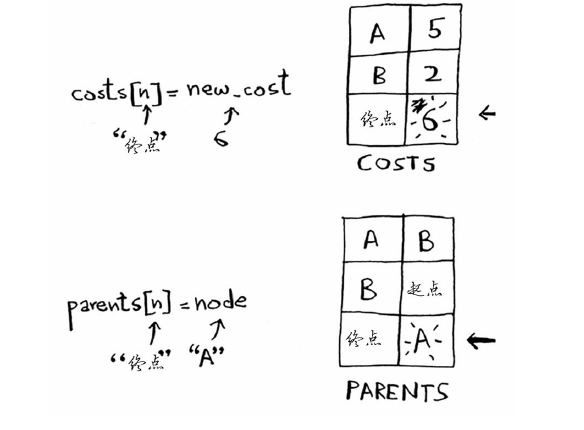def find_lowest_cost_node(costs):
lowest_cost = float("inf")
lowest_cost_node = None
for node in costs:  #遍历所有节点
cost = costs[node]
if cost < lowest_cost and node not in processed: #如果当前节点的开销更低且未处理过，就将其视为开销最低的节点
lowest_cost = costs
lowest_cost_node = node
return lowest_cost_node

7.6 小结

©️2019 CSDN 皮肤主题: 大白 设计师: CSDN官方博客